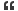1. ## c program

int z, x = 5, y = -10, a = 4, b = 2;
z = x++ - --y * b / a; what number will z in the sample code above contain?
a) 5
b) 6
c) 10
d) 11
e) 12Reply With Quote

2. ## Re: c program

Remember the following:

• The expression x++ evaluates to the current value of x, which is 5;
• The expression --y evaluates to the current value of y - 1, which is -11;
• The expression --y * b / a will be evaluated as (--y * b) / a since multiplication and division have the same precedence, so they are applied left to right;
• Since y, b, and a are all integers, the result of the division will be an integer value

Given all that, the answer should be obvious.Reply With Quote

3. ## Re: c program

So the result will be 5 - (-11) * 2 / 4 = 10.5 ans assuming that the value is integer we get 10Reply With Quote

4. ## Re: c programOriginally Posted by rumaxSo the result will be 5 - (-11) * 2 / 4 = 10.5 ans assuming that the value is integer we get 10
Basically, it will evaluate as
Code:
```   5 - ((-11 * 2) / 4)
== 5 - (-22       / 4)
== 5 - (-5           )
== 10```Reply With Quote

5. ## Re: c program

ans will be 10Reply With Quote

6. ## Re: c program

precedence of Unary operators are higher than Arithmatic operators.
x++ and --y are unary operators so these are evaluated first
then multiplication is performed ,
then division is performed ,
then subtraction is performed.
So the output is 10Reply With Quote

7. ## Re: c program

Nice Question....................

I tryed But i do not know it is correct or not

first priority gives to / after * +-from lerft to right

z=5++ + --10 * 2/4
=5++ + -11 *1/2
5++-5

i tryed my level best

Good QuestionReply With Quote

8. ## Re: c program

first Precedence is for / na from left to right
so first 2/4 operation will perform

check it once
good workReply With Quote

9.

####Posting Permissions

• You may not post new threads
• You may not post replies
• You may not post attachments
• You may not edit your posts
•